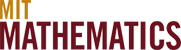Mathematical Logic & Foundations

Mathematical logic investigates the power of mathematical reasoning itself. The various subfields of this area are connected through their study of foundational notions: sets, proof, computation, and models. The period from the 1930s thru the 1970s saw great progress in logic. MIT was a major center in the field from the 1950s through the 1980s.

The exciting and active areas of logic today are set theory, model theory and connections with computer science. Set theory addresses various ways to axiomatize mathematics, with implications for understanding the properties of sets having large infinite cardinalities and connections with the axiomatization of mathematics. Model theory investigates particular mathematical theories such as complex algebraic geometry, and has been used to settle open questions in these areas. Theoretical computer science developed partially out of logic, and questions such as P =? NP are being pursued with techniques from logic.

Bjorn Poonen